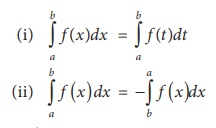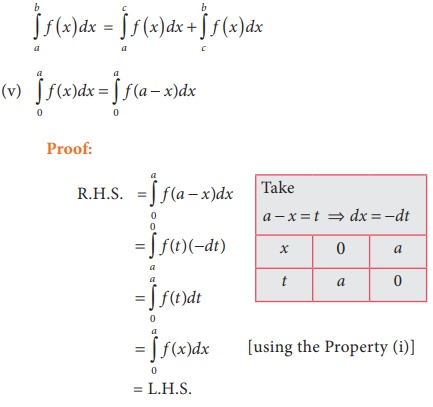Home | | Business Maths 12th Std | Properties of definite integrals

# Properties of definite integrals

Maths: Integral Calculus: Definite integrals: Properties of definite integrals

Properties of definite integrals(iii) If f (x) and g (x) are continuous functions in [a ,b] , then(iv) If  f (x) is a continuous function in [a ,band a < c < b, thenStudy Material, Lecturing Notes, Assignment, Reference, Wiki description explanation, brief detail
12th Business Maths and Statistics : Chapter 2 : Integral Calculus - I : Properties of definite integrals |